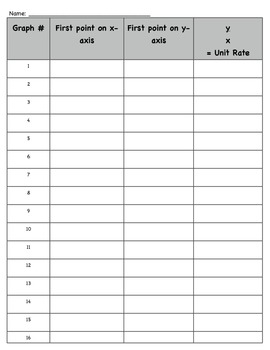# Finding the Unit Rate from a Graph 7.RP.2b, 7.RP.2dSubject
Resource Type
File Type

PDF

(4 MB|7 pages)
Standards
• Product Description
• StandardsNEW
This activity will help students master finding the unit rate from a graph. Students will have to find the point (1,r), which represents the unit rate. If there is no point (1,r), students will calculate the unit rate.

This aligns with the common core mathematics standards and to Module 1, The Story of Ratios.
Explain what a point (𝘹, 𝘺) on the graph of a proportional relationship means in terms of the situation, with special attention to the points (0, 0) and (1, 𝘳) where 𝘳 is the unit rate.
Identify the constant of proportionality (unit rate) in tables, graphs, equations, diagrams, and verbal descriptions of proportional relationships.
Total Pages
7 pages
N/A
Teaching Duration
N/A
Report this Resource to TpT
Reported resources will be reviewed by our team. Report this resource to let us know if this resource violates TpT’s content guidelines.